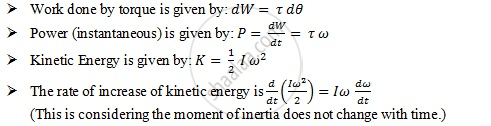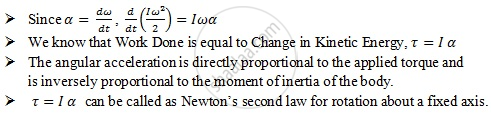Department of Pre-University Education, KarnatakaPUC Karnataka Science Class 11

# Dynamics of Rotational Motion About a Fixed Axis

## Notes

Dynamics of Rotational Motion about a Fixed Axis:

• Only those components of torques, which are along the direction of the fixed axis, need to be considered because the component of the torque perpendicular to the axis of rotation will tend to turn the axis from its position.

• This means

• We need to consider only those forces that lie in planes perpendicular to the axis. Forces which are parallel to the axis will give torques perpendicular to the axis.

• We need to consider only those components of the position vectors which are perpendicular to the axis. Components of position vectors along the axis will result in torques perpendicular to the axis.Sr no. Linear Motion Rotational Motion about a Fixed Axis 1 Displacement x Angular displacement θ 2 Velocity v = dx/dt Angular velocity ω = "dθ" /dt 3 Acceleration a = (dv)/dt Angular acceleration α = (dω) /dt 4 Mass M Moment of inertia I 5 Force F = Ma Torque tau = Ialpha 6 Work dW = Fds Work W =taud theta 7 Kinetic energy "K" = ("Mv"^2)/2 Kinetic energy K = (I ω^2)/2 8 Power P = F v Power P = τω 9 Linear momentum p = Mv Angular momentum L = I ω

If you would like to contribute notes or other learning material, please submit them using the button below.